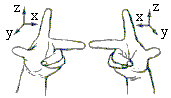# Multiplication of Vectors

This combination of words "multiplication" and "vector" appears in at least four circumstances:

of which only the fourth may be looked at as a (semi)group operation. Although the rest are also important, here I'll discuss only the latter. The vector multiplication (product) is defined for 3-dimensional vectors. To proceed, we need the notion of right- and left-handedness which apply to three mutually perpendicular vectors.Two noncollinear (non-parallel) vectors define a plane, and there are two ways to erect a third vector perpendicular to that plane (and, hence, to the two given vectors.) They are distinguished by the right- or left-handed rules. The direction defined by the right-handed rule is customarily preferred to the other one. When one looks from the top of the forefinger (z) the motion from the middle finger (x) towards the thump (y) is positive (counterclockwise).

The late Isaac Isimov once suggested apprehensively that technological advances may lead to thesaurus changes that would eliminate such dear to the heart notions as the clockwise and counterclockwise directions. Luckily, no technological progress could possibly affect the physical underpinning of the right-handed rule.

## Definition

Let a and b be two noncollinear vectors. Their cross (or external, or vector) product is defined as a vector a×b perpendicular to both a and b and whose

• direction is such that the three vectors a, b, and a×b form a right-handed system.
• length equals the area of the parallelogram built on the vectors a and b.

Cross product of collinear vectors is defined as 0. (Which is consistent with the noncollinear case as we may think of two parallel vectors as defining a (one line) parallelogram with zero area.Obviously the product has no unit element. One the positive side, both the associative and distributive laws hold. For the latter, it's obvious from the geometric considerations. The distributive law implies homogeneity (provided, of course, we first establish some kind of continuity. But this is feasible: small changes in either a or b result in small changes of the area of the parallelogram they define. The plane does not change drastically either.) For a scalar t,

 (ta)×b = a×(tb) = t(a×b)

The cross product is also anticommutative:

 a×b = - b×a

as it follows from the definition.

The cross product can be expressed in terms of a 3×3 determinant. Let e1, e2, and e3 be three mutually orthogonal unit vectors that form a right-handed system. Then, again by definition,

 e3 = e1×e2, e2 = e3×e1, e1 = e2×e3

If a = a1e1 + a2e2 + a3e3 and b = b1e1 + b2e2 + b3e3 then

 a×b = (a2b3 - a3b2)e1 - (a1b3 - a3b1)e2 + (a1b2 - a2b1)e3

which is often written asThe associative law does not hold for the cross product. I.e., in general,

 a×(b×c) ≠ (a×b)×c.

For example,

 e1×(e1×e2) = e1×e3 = -e2

whereas

 (e1×e1)×e2 = 0×e2 = 0.

To compensate, there are other useful properties, e.g.,

 a×(b×c) = (a·c)b - (a·b)c.

Especially useful is the mixed product of three vectors:

 a·(b×c) = det(a b c),

where the dot denotes the scalar product and the determinant det(a b c) has vectors a, b, c as its columns. The determinant equals the volume of the parallelepiped formed by the three vectors.### What Can Be Multiplied?